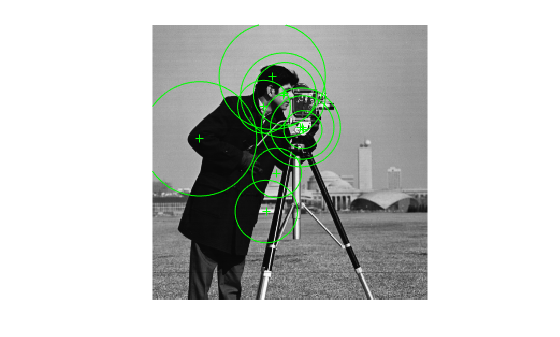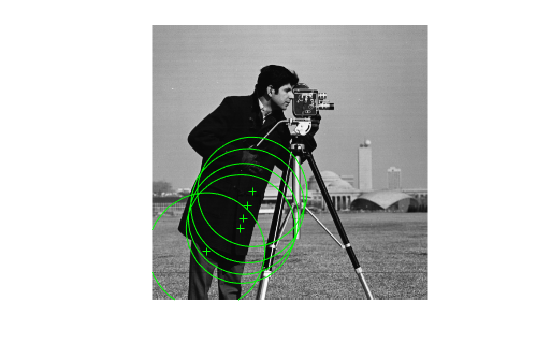# KAZEPoints

Object for storing KAZE interest points

## Description

This object provides the ability to pass data between the `detectKAZEFeatures` and `extractFeatures` functions. You can also use this object to manipulate and plot the data returned by these functions.Use the object to fill points interactively, where you might want to mix a non-KAZE interest oint detector with a KAZE descriptor.

## Creation

### Syntax

``points = KAZEPoints(location)``
``points = KAZEPoints(location,Name,Value)``

### Description

example

````points = KAZEPoints(location)` constructs a `KAZEPoints` object from an M-by-2 array [x y] of location coordinates. The scalar KAZEPoints object contains many points. Therefore `numel``(KAZEPoints)` always returns `1`. This value can be different than the result of `length(KAZEPoints)`, which returns the true number of points contained in the object.```

example

````points = KAZEPoints(location,Name,Value)` sets properties using one or more name-value pairs. Enclose each property name in quotes. For example, ```points = KAZEPoints('Metric',0.0)``````

### Input Arguments

expand all

Location of points, specified as an M-by-2 array of [x y] coordinates.

## Properties

expand all

Location of keypoints, specified as an M-by-2 matrix. Each row is of the form [x y] and represents the location of a keypoint. M is the number of keypoints. You cannot set this property, use the `location` input argument instead.

Number of keypoints held by the object, specified as a nonnegative integer.

Scale, specified as a scalar. The scale sets the size at which the interest points are detected.

Strength of response for the detected points, specified as a numeric value. The KAZE algorithm uses a determinant of an approximated Hessian.

Orientation of the detected feature, specified as an angle in radians. The angle is measured from the x-axis with the origin set by the `location` input. The `extractFeatures` function sets this property. Do not set it manually.

## Object Functions

 `isempty` Determine if points object is empty `length` Number of stored points `plot` Plot points `selectStrongest` Select points with strongest metrics `size` Return size of points object `selectUniform` Select uniformly distributed subset of feature points

## Examples

collapse all

Detect KAZE features and display 10 strongest points.

`I = imread('cameraman.tif');`

Detect KAZE features in the image.

`points = detectKAZEFeatures(I);`

Select the 10 strongest points.

`strongest = selectStrongest(points,10);`

Display the selected points.

``` imshow(I); hold on;```

Display the location and scale. The size of the circles displayed relate to the scale.

```plot(strongest); hold on;```Display the [x y] coordinates for the strongest points in the MATLAB Command Window.

`strongest.Location`
```ans = 10x2 single matrix 138.5041 95.8063 139.9253 95.8802 111.8975 48.2950 106.4036 174.1800 44.3964 106.4899 122.0368 65.9064 116.2702 138.2877 123.6542 64.7193 104.2719 76.5821 140.6228 97.9271 ```

Detect KAZE features and display set the specific KAZE points you want to plot.

`I = imread('cameraman.tif');`

Detect KAZE features in the image.

`points = detectKAZEFeatures(I);`

Select and display the last 5 points detected.

```imshow(I); hold on; plot(points(end-4:end)); hold off;```## Extended Capabilities

Introduced in R2017b